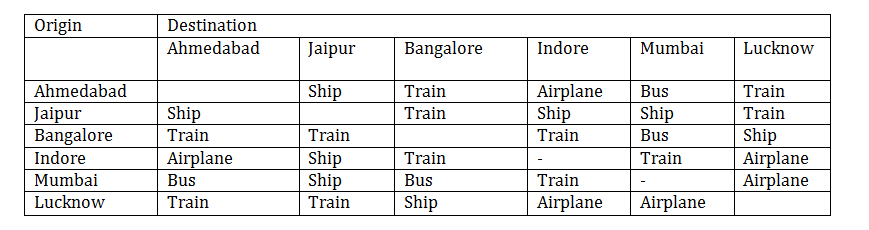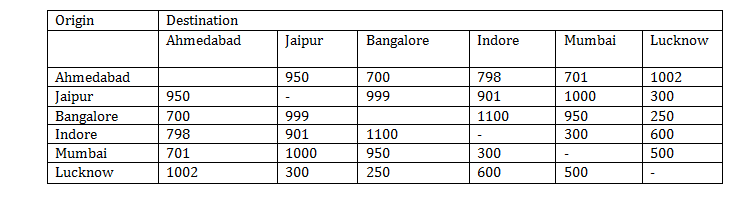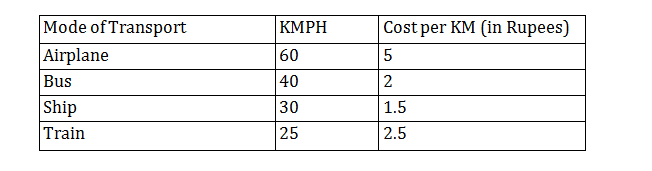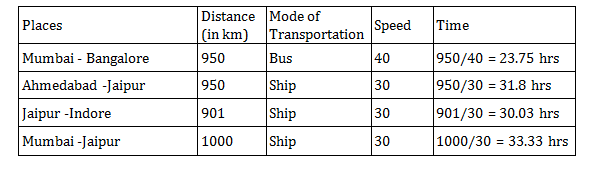Directions for questions 1 to 5: Read the following information and Tables and answer the questions.
Ahmedabad, Jaipur, Bangalore, Indore, Mumbai and Lucknow are 6 major Indian cities. For some reason people use only a certain mode of transport between a pair of cities. The modes of transport are provided in Table 1, while in Table 2 the distances between different pairs of cities are given. Table 3 provides the speed of the mode of transport and the cost associated with each of them.

Table 1: Mode of Transport between CitiesTable 2: Distance between Cities (KM)Table 3: Mode of Transport and CostQuestion 1 :  Mr. Ranjith lives in Mumbai and wants to travel to Indore. However, the train services are on halt due to laying of track for bullet trains across the country. In this scenario, which of the following is the least cost route to reach Indore?
B. Mumbai – Jaipur – Indore
C. Mumbai – Bangalore – Indore
D. Mumbai – Lucknow- Indore

Question 2 :  For which of the following options, travel time is the least?
A. Mumbai – Bangalore
C. Jaipur-Indore
D. Mumbai-Jaipur

Question 3 :  What is the least cost way to reach to Lucknow from Indore?
A. Take a flight from Indore to Lucknow
B. Take a ship from Indore to Jaipur and then take a train to Lucknow
C. Take a train from Indore to Bangalore and then take a ship to Lucknow
D. Take a train from Indore to Mumbai and then take a flight to Lucknow

Question 4 :  A school in Jaipur is planning for an excursion tour for its students. They want to show them Bangalore, Lucknow, and Ahmedabad, not necessarily in the same order. What is the minimum travel cost (in Rs.) the school should charge from each of the student for the entire tour?
A. Rs. 4300
B. Rs. 5000
C. Rs. 7500
D. Rs. 6800

Question 5 :  Which of the following cities can be reached from Ahmedabad in least time?
A. Jaipur
B. Bangalore
C. Mumbai
D. Lucknow

Answer 1 : (B)

Costs of each route given in the options is as follows:

Mumbai – Ahmedabad (Bus) and   Ahmedabad – Indore (Airplane)

Total fare = 701 × 2 + 798 × 5 = 1402 + 3990 = 5392

Mumbai – Jaipur (Ship) and Jaipur – Indore (ship)

Total fare = 1000 × 1.5 + 901 × 1.5 = 1500 + 1351.5 = 2851.5

Mumbai – Bangalore (Bus) and Bangalore – Indore (Train)

Total = 950 × 2 +1100 × 2.5 = 1900 + 2750 = 4650

Mumbai – Lucknow (Airplane) and Lucknow – Indore (Airplane)

Total fare = 500 × 5 + 600 × 5 = 5500

Answer 2 : (A)

Time taken in each of the given options case is shown below:Answer 3 : (B)

The costs for each of the given route is shown below.

Indore – Lucknow = 600 × 5 = Rs. 3000.

Indore – Jaipur – Lucknow = 901 × 1.5 + 300 × 2.5 = Rs. 2101.5.

Indore – Bangalore – Lucknow = 1100 × 2.5 + 250 × 1.5 = Rs. 3125.

Indore – Mumbai – Lucknow = 300 × 2.5 + 500 × 5 = Rs. 3250.

Answer 4 : (A)

The cost will be minimum through the given route.

Bangalore Lucknow Jaipur

Hence, the required cost (in Rs.)

= (950 + 250) × 1. 5 + (700 + 300) × 2.5 = 4300.

Answer 5 : (C)

Time to reach each of the cities is shown below.

Ahmedabad – Jaipur (by Ship) = 950/30 = 31.67 hrs

Ahmedabad – Bangalore (by Train) = 700 / 25 = 28 hrs

Ahmedabad – Mumbai (by Bus) = 701 /40= 17.525 hrs

Ahmedabad – Lucknow (by Train) = 1002/25 = 40.08 hrs